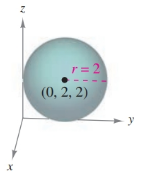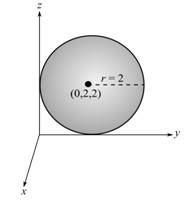Chapter 7.1, Problem 29E### Calculus: An Applied Approach (Min...

10th Edition
Ron Larson
ISBN: 9781305860919

#### Solutions

Chapter
Section### Calculus: An Applied Approach (Min...

10th Edition
Ron Larson
ISBN: 9781305860919
Textbook Problem
1 views

# Finding the equation of a Sphere In Exercises 29–38, find the standard equation of the sphere with the given characteristics. See Examples 4 and 5.To determine

To calculate: The standard equation of sphere from the provided figure,Explanation

Given Information:

The provided figure,

Formula used:

The standard equation of a sphere with centre at (h,k,l) and radius r is,

(xh)2+(yk)2+(zj)2=r2

Calculation:

From the provided figure, the centre of sphere is (0,2,2) and radius is 2.

The standard equation of a sphere,

(xh)2+(yk)2+

### Still sussing out bartleby?

Check out a sample textbook solution.

See a sample solution

#### The Solution to Your Study Problems

Bartleby provides explanations to thousands of textbook problems written by our experts, many with advanced degrees!

Get Started

#### For Problems 11-18, evaluate each expression. (2.91)53.295

Mathematical Applications for the Management, Life, and Social Sciences

#### True or False: If , then converge absolutely.

Study Guide for Stewart's Single Variable Calculus: Early Transcendentals, 8th

#### What are the five steps of the scientific method?

Research Methods for the Behavioral Sciences (MindTap Course List)